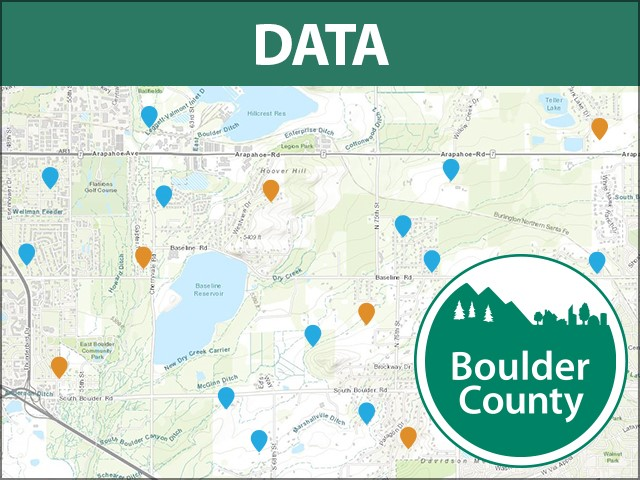### Description

more
A plat as defined in Article 18-184A as map of certain described subdivided land, including supporting materials, which constitutes an instrument for recording real estate interests, is prepared and approved in accordance with the Subdivision Regulations, and is recorded in the office of the County Clerk and Recorder. The term "plat," as used in this Code as a reference to subdivided land, does not include any map, depiction, or document of any kind describing unsubdivided land, even though the term "plat" may be used in such document (for example, a document denominated as a "survey plat" or "subdivision exemption plat" which does not describe divisions of land approved pursuant to the Subdivision Regulations). Users must always check the recorded plat, resolution, and development agreement for conditions of approval.

### Dataset Attributes

• OBJECTID
Number
• SUBCODE
Text
{"value"=>"8294", "count"=>18} (), {"value"=>"6461", "count"=>10} (), {"value"=>" ", "count"=>9} (), {"value"=>"3087", "count"=>7} (), {"value"=>"7044", "count"=>5} (), {"value"=>"909", "count"=>4} (), {"value"=>"7340", "count"=>4} (), {"value"=>"3508", "count"=>4} (), {"value"=>"2670", "count"=>4} (), {"value"=>"2493", "count"=>4} ()
• REC_DATE
Date
-2990304000000 to 1582588800000
• NAME_ALL
Text
{"value"=>"MOUNTAIN VIEW ESTATES NUPUD", "count"=>3} (), {"value"=>"CRYSTAL VIEWS NUPUD", "count"=>3} (), {"value"=>"YELLOWSTONE VISTAS 1 NUPUD", "count"=>2} (), {"value"=>"YELLOWSTONE FARMS NUPUD", "count"=>2} (), {"value"=>"YELLOWSTONE ESTATES NUPUD", "count"=>2} (), {"value"=>"WOODLAND VILLAGE NUPUD", "count"=>2} (), {"value"=>"WOOD MEADOW NCNUPUD CORRECTION PLAT", "count"=>2} (), {"value"=>"WINTERVIEW PUD", "count"=>2} (), {"value"=>"WINDRIDGE NUPUD", "count"=>2} (), {"value"=>"WILLOWS", "count"=>2} ()
• LUNUM
Number
• TYPE
Text
{"value"=>"SUB", "count"=>486} (), {"value"=>"NUPUD", "count"=>346} (), {"value"=>"PUD", "count"=>218} (), {"value"=>"TOWNSITE", "count"=>70} (), {"value"=>"EXEMPT", "count"=>43} (), {"value"=>"TDRPUD", "count"=>32} (), {"value"=>"NCNUPUD", "count"=>13} ()
• LOTTYPE
Text
{"value"=>"LOT", "count"=>916} (), {"value"=>"OUTLOT", "count"=>291} ()
• REC_NUM
Text
{"value"=>"0", "count"=>43} (), {"value"=>"902381", "count"=>4} (), {"value"=>"449038", "count"=>4} (), {"value"=>"1530701", "count"=>4} (), {"value"=>"90865622", "count"=>3} (), {"value"=>"90828883", "count"=>3} (), {"value"=>"90781026", "count"=>3} (), {"value"=>"90730570", "count"=>3} (), {"value"=>"408340", "count"=>3} (), {"value"=>"2462452", "count"=>3} ()
• GlobalID
Number
• Sanitary System
Text
{"value"=>"Septic", "count"=>798} (), {"value"=>"Sewer", "count"=>394} (), {"value"=>"No Homes", "count"=>7} (), {"value"=>"Nederland", "count"=>4} (), {"value"=>"Unknown", "count"=>3} (), {"value"=>"Ward", "count"=>2} ()
• HOA ARC Referral
Text
{"value"=>"No", "count"=>1137} (), {"value"=>"Yes", "count"=>71} ()
• Landscape_Req
Text
{"value"=>"No", "count"=>1134} (), {"value"=>"Yes", "count"=>74} ()
• CO Condition
Text
{"value"=>"No", "count"=>1175} (), {"value"=>"Yes", "count"=>33} ()
• SPR_Exempt
Text
{"value"=>"No", "count"=>1091} (), {"value"=>"Yes", "count"=>117} ()
• Notes
Text
{"value"=>"Height, Envelope", "count"=>18} (), {"value"=>"TDC > 9,000 sf", "count"=>9} (), {"value"=>"Height", "count"=>8} (), {"value"=>"Envelope, Height", "count"=>7} (), {"value"=>"Size, Height, Envelope", "count"=>6} (), {"value"=>"Height, Size", "count"=>6} (), {"value"=>"Envelope, Height, Setbacks", "count"=>6} (), {"value"=>"Height, Outlot Usage Restrictions", "count"=>4} (), {"value"=>"Height, Envelope, Outlot Usage, Homestead", "count"=>3} (), {"value"=>"Size, Homestead, Outlot usage", "count"=>2} ()
• Shape
Number
• SHAPE.area
Number
• SHAPE.len
Number

• By on March 11, 2016
• Updated 9 months ago
• Peak To Peak Scenic Corridor• Zoning - Zoning Districts• Marijuana Business Regulatory Areas• Mobile Homes